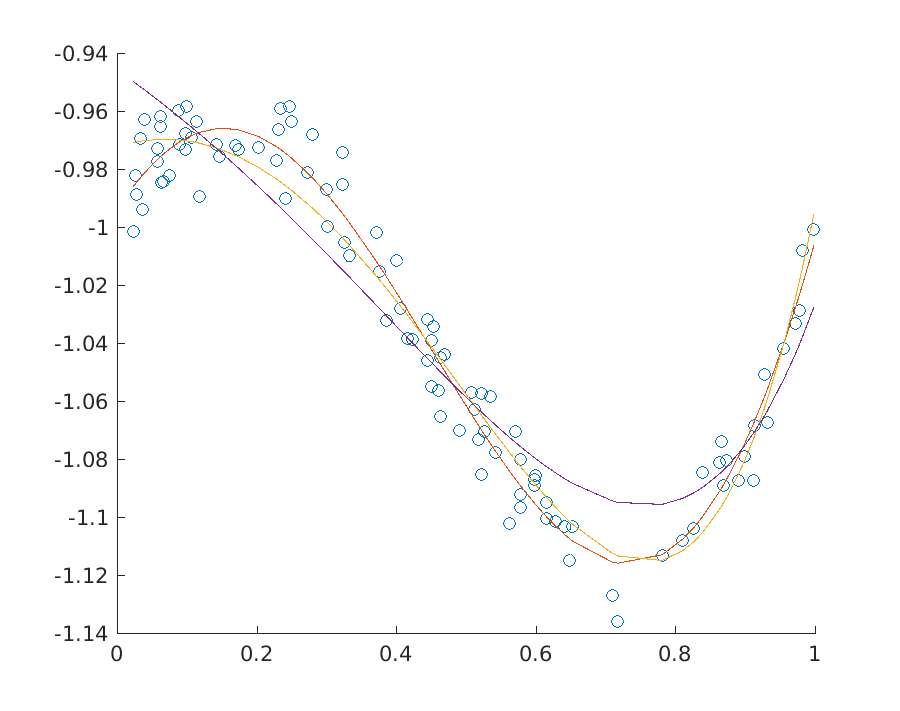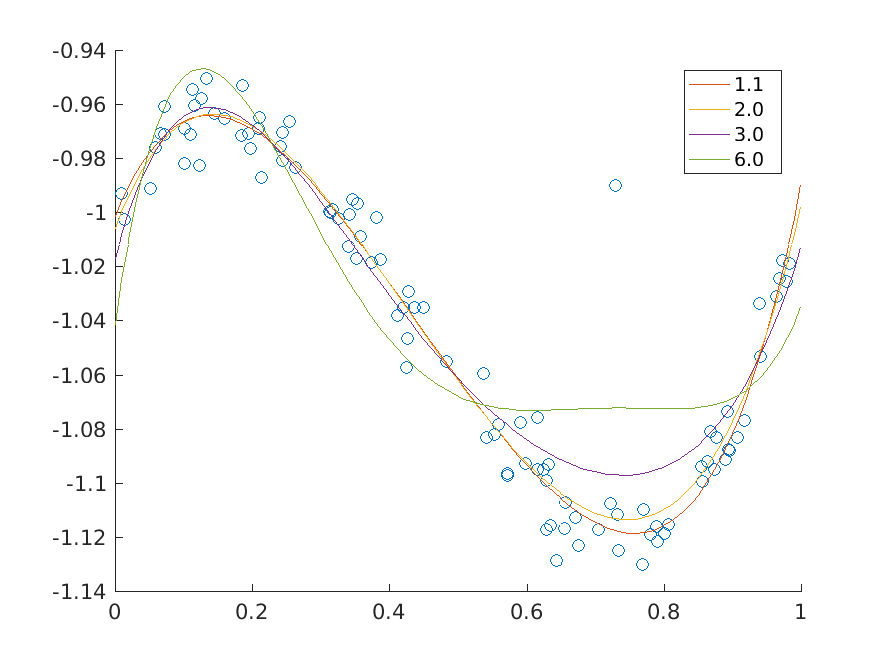# 10.2 Least Squares and Other Norm Minimization Problems¶

A frequently occurring problem in statistics and in many other areas of science is a norm minimization problem

(10.13)$\begin{split}\begin{array}{ll} \mbox{minimize} & \|F x - g\|,\\ \mbox{subject to} & Ax=b, \end{array}\end{split}$

where $$x\in\real^n$$ and of course we can allow other types of constraints. The objective can involve various norms: infinity norm, 1-norm, 2-norm, $$p$$-norms and so on. For instance the most popular case of the 2-norm corresponds to the least squares linear regression, since it is equivalent to minimization of $$\|Fx-g\|_2^2$$.

## 10.2.1 Least squares, 2-norm¶

In the case of the 2-norm we specify the problem directly in conic quadratic form

(10.14)$\begin{split}\begin{array}{ll} \mbox{minimize} & t,\\ \mbox{subject to} & (t, Fx-g)\in\Q^{k+1}, \\ & Ax=b. \end{array}\end{split}$

The first constraint of the problem can be represented as an affine conic constraint. This leads to the following model.

Listing 10.8 Script solving problem (10.14) Click here to download.
# Least squares regression
# minimize \|Fx-g\|_2
norm_lse <- function(F,g,A,b)
{
n <- dim(F)
k <- length(g)
m <- dim(A)

# Linear constraints in [x; t]
prob <- list(sense="min")
prob$A <- cbind(A, rep(0, m)) prob$bx <- rbind(rep(-Inf, n+1), rep(Inf, n+1))
prob$bc <- rbind(b, b) prob$c <- c(rep(0, n), 1)

# Affine conic constraint
prob$F <- rbind( c(rep(0,n), 1), cbind(F, rep(0, k)) ) prob$g <- c(0, -g)
prob$cones <- matrix(list("QUAD", k+1, NULL)) rownames(prob$cones) <- c("type","dim","conepar")

# Solve
r <- mosek(prob, list(verbose=1))
stopifnot(identical(r$response$code, 0))
r$sol$itr$xx[1:n] }  ## 10.2.2 Ridge regularisation¶ Regularisation is classically applied to reduce the impact of outliers and to control overfitting. In the conic version of ridge (Tychonov) regression we consider the problem (10.15)$\begin{split}\begin{array}{ll} \mbox{minimize} & \|F x - g\|_2+ \gamma\|x\|_2,\\ \mbox{subject to} & Ax=b, \end{array}\end{split}$ which can be written explicitly as (10.16)$\begin{split}\begin{array}{ll} \mbox{minimize} & t_1+\gamma t_2,\\ \mbox{subject to} & (t_1, Fx-g)\in\Q^{k+1}, \\ & (t_2, x)\in\Q^{n+1},\\ & Ax=b. \end{array}\end{split}$ The implementation is a small extension of that from the previous section. Listing 10.9 Script solving problem (10.16) Click here to download. # Least squares regression with regularization # minimize \|Fx-g\|_2 + gamma*\|x\|_2 norm_lse_reg <- function(F,g,A,b,gamma) { n <- dim(F) k <- length(g) m <- dim(A) # Linear constraints in [x; t1; t2] prob <- list(sense="min") prob$A <- cbind(A, rep(0, m), rep(0, m))
prob$bx <- rbind(rep(-Inf, n+2), rep(Inf, n+2)) prob$bc <- rbind(b, b)
prob$c <- c(rep(0, n), 1, gamma) # Affine conic constraint prob$F <- rbind(     c(rep(0,n), 1,         0),
cbind(F,        rep(0, k), rep(0, k)),
c(rep(0,n), 0,         1),
cbind(diag(n),  rep(0, n), rep(0, n)))
prob$g <- c(0, -g, rep(0, n+1)) prob$cones <- cbind(matrix(list("QUAD", k+1, NULL)),
rownames(prob$cones) <- c("type","dim","conepar") # Solve r <- mosek(prob, list(verbose=1)) stopifnot(identical(r$response$code, 0)) r$sol$itr$xx[1:n]
}


Note that classically least squares problems are formulated as quadratic problems and then the objective function would be written as

$\|Fx-g\|_2^2+\gamma\|x\|_2^2.$

This version can easily be obtained by replacing the quadratic cone with an appropriate rotated quadratic cone in (10.16). Then they core of the implementation would change as follows:

Listing 10.10 Script solving classical quadratic ridge regression Click here to download.
    # Affine conic constraint
prob$F <- rbind( c(rep(0,n), 1, 0), c(rep(0, n+2)), cbind(F, rep(0, k), rep(0, k)), c(rep(0,n), 0, 1), c(rep(0, n+2)), cbind(diag(n), rep(0, n), rep(0, n))) prob$g <- c(0, 0.5, -g, 0, 0.5, rep(0, n))
prob$cones <- cbind(matrix(list("RQUAD", k+2, NULL)), matrix(list("RQUAD", n+2, NULL))) rownames(prob$cones) <- c("type","dim","conepar")


Fig. 10.2 shows the solution to a polynomial fitting problem for a few variants of least squares regression with and without ridge regularization.Fig. 10.2 Three fits to a dataset at various levels of regularization.¶

## 10.2.3 Lasso regularization¶

In lasso or least absolute shrinkage and selection operator the regularization term is the 1-norm of the solution

(10.17)$\begin{split}\begin{array}{ll} \mbox{minimize} & \|F x - g\|_2+ \gamma\|x\|_1,\\ \mbox{subject to} & Ax=b. \end{array}\end{split}$

This variant typically tends to give preference to sparser solutions, i.e. solutions where only a few elements of $$x$$ are nonzero, and therefore it is used as an efficient approximation to the cardinality constrained problem with an upper bound on the 0-norm of $$x$$. To see how it works we first implement (10.17) adding the constraint $$t\geq \|x\|_1$$ as a series of linear constraints

$u_i\geq -x_i,\ u_i\geq x_i,\ t\geq \sum u_i,$

so that eventually the problem becomes

$\begin{split}\begin{array}{ll} \mbox{minimize} & t_1+\gamma t_2,\\ \mbox{subject to} & u+x\geq 0,\\ & u-x\geq 0,\\ & t_2-e^Tu \geq 0,\\ & Ax=b,\\ & (t_1, Fx-g) \in \Q^{k+1}. \end{array}\end{split}$
Listing 10.11 Script solving problem (10.17) Click here to download.
# Least squares regression with lasso regularization
# minimize \|Fx-g\|_2 + gamma*\|x\|_1
norm_lse_lasso <- function(F,g,A,b,gamma)
{
n <- dim(F)
k <- length(g)
m <- dim(A)

# Linear constraints in [x; u; t1; t2]
prob <- list(sense="min")
prob$A <- rbind(cbind(A, matrix(0, m, n+2)), cbind(diag(n), diag(n), matrix(0, n, 2)), cbind(-diag(n), diag(n), matrix(0, n, 2)), c(rep(0, n), rep(-1, n), 0, 1)) prob$bx <- rbind(rep(-Inf, 2*n+2), rep(Inf, 2*n+2))
prob$bc <- rbind(c(b, rep(0, 2*n+1)), c(b, rep(Inf, 2*n+1))) prob$c <- c(rep(0, 2*n), 1, gamma)

# Affine conic constraint
prob$F <- rbind(c(rep(0, 2*n), 1, 0), cbind(F, matrix(0, k, n+2))) prob$g <- c(0, -g)
prob$cones <- matrix(list("QUAD", k+1, NULL)) rownames(prob$cones) <- c("type","dim","conepar")

# Solve
r <- mosek(prob, list(verbose=1))
stopifnot(identical(r$response$code, 0))
r$sol$itr$xx[1:n] }  The sparsity pattern of the solution of a large random regression problem can look for example as follows: Lasso regularization Gamma 0.0100 density 99% |Fx-g|_2: 54.3722 Gamma 0.1000 density 87% |Fx-g|_2: 54.3939 Gamma 0.3000 density 67% |Fx-g|_2: 54.5319 Gamma 0.6000 density 40% |Fx-g|_2: 54.8379 Gamma 0.9000 density 26% |Fx-g|_2: 55.0720 Gamma 1.3000 density 12% |Fx-g|_2: 55.1903  ## 10.2.4 p-norm minimization¶ Now we consider the minimization of the $$p$$-norm defined for $$p>1$$ as (10.18)$\|y\|_p = \left(\sum_i |y_i|^p \right)^{1/p}.$ We have the optimization problem: (10.19)$\begin{split}\begin{array}{ll} \mbox{minimize} & \|F x - g\|_p,\\ \mbox{subject to} & Ax=b. \end{array}\end{split}$ Increasing the value of $$p$$ forces stronger penalization of outliers as ultimately, when $$p\to\infty$$, the $$p$$-norm $$\|y\|_p$$ converges to the infinity norm $$\|y\|_\infty$$ of $$y$$. According to the Modeling Cookbook the $$p$$-norm bound $$t\geq \|Fx-g\|_p$$ can be added to the model using a sequence of three-dimensional power cones and we obtain an equivalent problem (10.20)$\begin{split}\begin{array}{ll} \mbox{minimize} & t \\ \mbox{subject to} & (r_i,t,(Fx-g)_i)\in\POW_3^{1/p,1-1/p}, \\ & e^Tr = t, \\ & Ax = b. \end{array}\end{split}$ The power cones can be added one by one to the structure representing affine conic constraints. Each power cone will require one $$r_i$$, one copy of $$t$$ and one row from $$F$$ and $$g$$. An alternative solution is to create the vector $[r_1;\ldots;r_k;t;\ldots;t;Fx-g]$ and then reshuffle its elements into $[r_1;t;F_1x-g_1;\ldots;r_k;t;F_kx-g_k]$ using an appropriate permutation matrix. This approach is demonstrated in the code below. Listing 10.12 Script solving problem (10.20) Click here to download. # P-norm minimization # minimize \|Fx-g\|_p norm_p_norm <- function(F,g,A,b,p) { n <- dim(F) k <- length(g) m <- dim(A) # Linear constraints in [x; r; t] prob <- list(sense="min") prob$A <- rbind(cbind(A,    matrix(0, m, k+1)),
c(rep(0, n), rep(1, k), -1))
prob$bx <- rbind(rep(-Inf, n+k+1), rep(Inf, n+k+1)) prob$bc <- rbind(c(b, 0), c(b, 0))
prob$c <- c(rep(0, n+k), 1) # Permutation matrix which picks triples (r_i, t, F_ix-g_i) M <- cbind(sparseMatrix(seq(1,3*k,3), seq(1,k), x=rep(1,k), dims=c(3*k,k)), sparseMatrix(seq(2,3*k,3), seq(1,k), x=rep(1,k), dims=c(3*k,k)), sparseMatrix(seq(3,3*k,3), seq(1,k), x=rep(1,k), dims=c(3*k,k))) # Affine conic constraint prob$F <- M %*% rbind(cbind(matrix(0, k, n), diag(k), rep(0, k)),
cbind(matrix(0, k, n+k), rep(1, k)),
cbind(F, matrix(0, k, k+1)))
prob$g <- as.numeric(M %*% c(rep(0, 2*k), -g)) prob$cones <- matrix(list("PPOW", 3, c(1, p-1)), nrow=3, ncol=k)
rownames(prob$cones) <- c("type","dim","conepar") # Solve r <- mosek(prob, list(verbose=1)) stopifnot(identical(r$sol$itr$prosta, "PRIMAL_AND_DUAL_FEASIBLE"))
r$sol$itr\$xx[1:n]
}Fig. 10.3 $$p$$-norm minimizing fits of a polynomial of degree at most 5 to the data for various values of $$p$$.¶